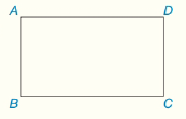Chapter 4.CT, Problem 11CTElementary Geometry For College St...

7th Edition
Alexander + 2 others
ISBN: 9781337614085

Solutions

Chapter
SectionElementary Geometry For College St...

7th Edition
Alexander + 2 others
ISBN: 9781337614085
Textbook Problem

In rectangle ABCD, AD=12 and DC=5. Find the length of diagonal A C ¯ (not shown)To determine

To Find:

Length of AC.

Explanation

Given:

ABCD is a rectangle and AD = 12 and DC = 5.

Calculation:

All interior angles in a rectangle are right angle.

D is a right angle and hence ΔACD is a right triangle with hypotenuse AC.

By Pythagorean Theorem in ΔACD,

AC2

Still sussing out bartleby?

Check out a sample textbook solution.

See a sample solution

The Solution to Your Study Problems

Bartleby provides explanations to thousands of textbook problems written by our experts, many with advanced degrees!

Get Started

In Exercises 1-6, solve for y. x3y+2y=1

Calculus: An Applied Approach (MindTap Course List)

let f(x) = x 1, g(x) = x+1, and h(x) = 2x3 1. Find the rule for each function. 13. gh

Applied Calculus for the Managerial, Life, and Social Sciences: A Brief Approach

The x-coordinate of the center of mass of the region bounded by , x = 1, x = 2, y = 0 is: ln 2 1 2 ln 2

Study Guide for Stewart's Single Variable Calculus: Early Transcendentals, 8th